GibbsSamplingIntro

Markov Chain Monte Carlo (MCMC) methods are a popular way of solving the math problems in Bayesian statistics.
Unfortunately, while popular packages for doing MCMC can be convenient, they can sometimes seem like opaque black boxes.

So, let’s write our own Gibbs samplers to have something to play with, and try to understand what is going on, and how popular packages like JAGS (Just Another Gibbs Sampler), NIMBLE, and BUGS (Bayesian inference Using Gibbs Sampling.

This code was writtenand run from a Jupyter notebook. You can copy and paste the code, or ask me for the ipynb file and install Jupyter notebook, and then from within R, run install.packages("IRkernel") and IRkernel::installspec(). Then you can launch the notebook (jupyter notebook from the command line) and run the file within a browser.

First, why MCMC? Integration is hard.
If you would like a Bayesian posterior, you need to solve

\begin{align}
\pi(\boldsymbol{\theta}|y) = \frac{ \pi(\boldsymbol{\theta})f(\textbf{y}|\boldsymbol{\theta}) }{ \int_{-\infty}^{\infty} \pi(\theta)f(\textbf{y}|\boldsymbol{\theta}) d\boldsymbol{\theta} }.
\end{align}

The integral in the denominator is normally intractable. Furthermore, if you want to answer useful questions with that posterior, you’ll often find yourself needing to solve problems like:

\begin{align}
0.025 &= \int_{-\infty}^l \int_{-\infty}^{\infty} \pi(\boldsymbol{\theta}|\textbf{y})d\boldsymbol{\theta}_{-k} d\theta_k\\
0.025 &= \int_u^{\infty} \int_{-\infty}^{\infty} \pi(\boldsymbol{\theta}|\textbf{y})d\boldsymbol{\theta}_{-k} d\theta_k
\end{align}

that is, marginalize out all parameters except $\theta_k$, and then solve for a (lower) $l$ bound of $\theta_k$ such that $2.5\%$ of the probability mass lies below it, and a similar upper bound, $u$.
That is a lot of integration.

We need numerical techniques. Markov Chain Monte Carlo techniques are among the best and the most flexible $-$ especially for high dimensional problems.
They let us draw samples from a posterior distribution. These samples give us an emperical cdf we can use to approximate the actual distribution. Like all numerical techniques, MCMC isn’t perfect and the results have error. But because the results are in the form of samples describing a population (the true posterior), we as statisticians are hopefully comfortable assessing how well that sample (MCMC samples) really describes the “population”.
Typically, we run the sampler long enough to get a big sample size and thus a good approximation.
Just make sure not to confuse the data samples and population, from the mcmc samples and population/true posterior. Running MCMC for longer isn’t going to help with noise from bad data.

So, what is Gibbs sampling?

Gibbs sampling, in a nutshell, lets you break up sampling from a complicated model into pieces. If the full parameter vector is $\boldsymbol{\theta}$, we’ll have $P$ pieces, $\boldsymbol{\theta}_p,p=1,\ldots,P$.

To sample $\boldsymbol{\theta}$, all you’ll have to do is sample from each $\boldsymbol{\theta}_p$, conditioning on the remaining parameters $\boldsymbol{\theta}_{-p}$.

We’ll use a superscript, $\boldsymbol{\theta}^i$, to indicate the mcmc sample number. So, say we have sample $\boldsymbol{\theta}^{i-1}$. Given this sample we can draw sample $\boldsymbol{\theta}^{i}$ by:

1. Sampling $\boldsymbol{\theta}_1$ conditioning on $\boldsymbol{\theta}_{-1}^{i-1}$
2. Sampling $\boldsymbol{\theta}_2$ conditioning on $\boldsymbol{\theta}_1^{i},\boldsymbol{\theta}_{3,\ldots,P}^{i-1}$
3. Sampling $\boldsymbol{\theta}_3$ conditioning on $\boldsymbol{\theta}_{1,2}^{i},\boldsymbol{\theta}_{4,\ldots,P}^{i-1}$
4. Sampling…

etc. If sample $i-1$ was from the posterior, then the $i$th will as well. However, the samples will not be iid, because they’re conditioned on previous observations. Additionally, that means if $\boldsymbol{\theta}^i$ isn’t representative of the posterior $-$ for example, perhaps because it was a randomly generated initial value you used to start the chain $-$ then $\boldsymbol{\theta}^{i+1}$ is not necessarilly either. It can take a while for the chain to move to representative, “valid” values. This is one reason why it is common to discard the early iterations as a “warmup”. Another reason is that sometimes models need to adapt.

Lets take a close look at a very simple example: the beta-binomial. The beta binomial is:
\begin{align*}
y &\sim \text{Binomial}(n, \theta)\\
\theta &\sim \text{Beta}(\alpha, \beta).
\end{align*}

What are the distributions of $y$ and $\theta$, given $n, \alpha,$ and $\beta$?
First of all, $f(y|n,\theta)$ is simply a Binomial distribtion. So to sample $y$, we sample from a Binomial$(n,\theta)$.

Slightly more difficult, we need the distribution of $\theta$ given $y$.
\begin{align*}
f(\theta | y) &\propto f(y | \theta) f(\theta)\\
&= \theta^{y}(1-\theta)^{n-y}\theta^{\alpha – 1}(1-\theta)^{n-y}\\
&= \theta^{\alpha + y – 1}(1-\theta)^{n – y + \beta – 1}.
\end{align*}
Therefore, we can recognize this as the kernel of a Beta$(\alpha + y, n – y + \beta)$ distribution.

So, to draw sample $y^i, \theta^i$ given $y^{i-1},\theta^{i-1}$, we’d only need to

1. sample $y^i$ from a Binomial$(n, \theta^{i-1})$.
2. sample $\theta^i$ from a Beta$(\alpha + y, n – y + \beta)$.

We can implement this with a simple for loop in R. To start this chain, we’ll randomly initialize $\theta$ with a sample from a random uniform. Sampling, using $\alpha = 3, \beta = 2$:

In :
rand_BetaBinomial <- function(iter, N, alpha, beta, warmup = 1000L, theta_init = runif(1)){
# We preallocate the theta and y vectors.
# "Preallocate" means to create the vectors ("allocating" their memory),
# so that we can fill them in as we go. That is much faster than repeating
# theta <- c(theta, new_sample)
# in a for loop.
theta <- double(iter)
y <- integer(iter)
# Now, we sample our first y from the initial value of theta
y <- rbinom(1,N, theta_init)
# Run a for loop for "warmup" iterations. Here, we're just overwriting our old values, instead of saving them.
for (i in 1:max(1L,warmup)){
theta <- rbeta(1, y + alpha, N - y + beta)
y <- rbinom(1, N, theta)
}
# Now we sample. Warmups were set to be at least 1, so that we can start our posterior sampling at 2.
# we want to start at 2, so that we can use "i-1", to reference the previous iteration.
for (i in 2:iter){
theta[i] <- rbeta(1, y[i-1] + alpha, N - y[i-1] + beta)
y[i] <- rbinom(1, N, theta[i])
}
data.frame(theta = theta, y = y)
}

set.seed(1)
system.time(beta_binom <- rand_BetaBinomial(1e5, 20, 3, 2))

   user  system elapsed
0.365   0.014   0.381 

Neat. We can get a hundred thousand samples in a negligible amount of practical time.
Let’s focus on $y$, because it has the beta-binomial distribution:

In :
library(tidyverse)
y_distribution <- beta_binom %>%
select(y) %>%
group_by(y) %>%
tally() %>%
mutate(MCMC = n / sum(n))
y_distribution

── Attaching packages ─────────────────────────────────────── tidyverse 1.2.1 ──
✔ ggplot2 3.1.0     ✔ purrr   0.2.5
✔ tibble  2.0.1     ✔ dplyr   0.7.8
✔ tidyr   0.8.2     ✔ stringr 1.3.1
✔ readr   1.3.1     ✔ forcats 0.3.0
── Conflicts ────────────────────────────────────────── tidyverse_conflicts() ──

y n MCMC
0 164 0.00164
1 516 0.00516
2 1039 0.01039
3 1698 0.01698
4 2402 0.02402
5 3258 0.03258
6 3903 0.03903
7 4815 0.04815
8 5587 0.05587
9 6204 0.06204
10 6848 0.06848
11 7368 0.07368
12 7746 0.07746
13 7837 0.07837
14 7993 0.07993
15 7644 0.07644
16 7179 0.07179
17 6374 0.06374
18 5313 0.05313
19 3927 0.03927
20 2185 0.02185
In :
beta_binom %>%
summarize(
mean_theta = mean(theta),
stdev_theta = sd(theta),
mean_y = mean(y),
stdev_y = sd(y)
)

mean_theta stdev_theta mean_y stdev_y
0.5995309 0.1992082 11.99333 4.458807

Lets compare these frequencies with the actual $pmf$ using dbetabinom from rmutil. That function uses the overdispersion parameterization, where

\begin{align*}
s &= \alpha + \beta\\
m &= \frac{\alpha}{\alpha + \beta}.
\end{align*}

so that, given $\alpha = 3, \beta = 2$, we have $s = 5, m = 0.6$.

In :
library(rmutil)
theme_set(theme_bw())
options(width = 150)

y_summary <- y_distribution  %>% mutate(
PMF = dbetabinom(y, 20, 0.6, 5)
) %>%
gather(estimate, probability, MCMC, PMF)

ggplot(y_summary) + geom_col(aes(y, probability, fill = estimate), position = position_dodge())

Attaching package: ‘rmutil’

The following object is masked from ‘package:tidyr’:

nesting

The following object is masked from ‘package:stats’:

nobs

The following objects are masked from ‘package:base’:

as.data.frame, units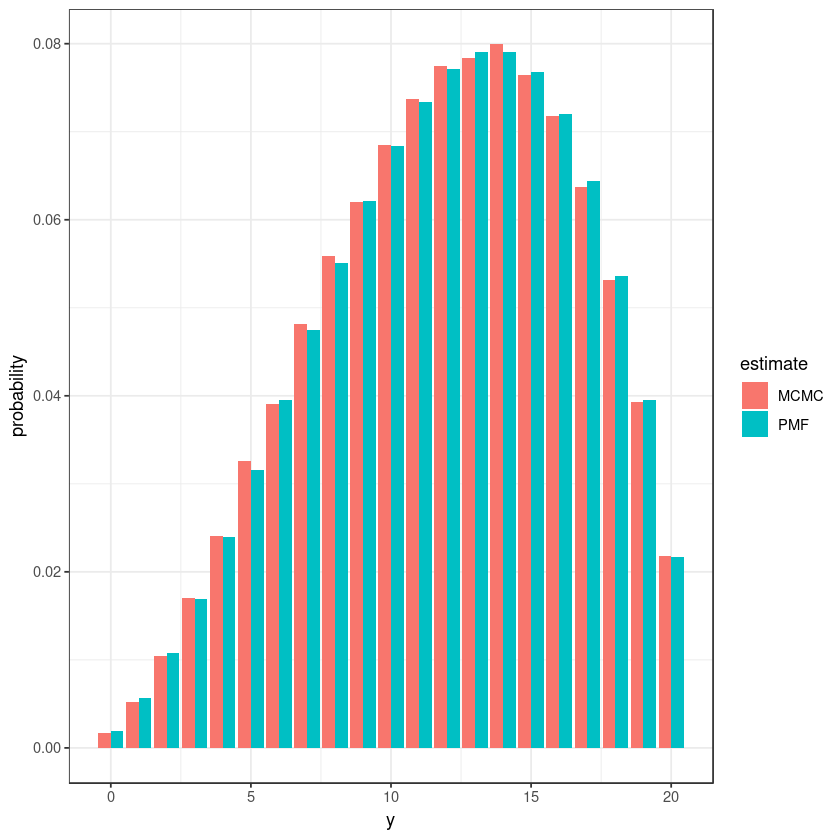Lets dive into this a little more. A familiar diagonostic is effective sample size.
A commonly used formula is $n_{eff} = \frac{n}{1 + 2\sum_{n=1}^{n-1}\rho_n}$.

The function acf starts with lag 0, where the autocorrelation is 1 (everything has a 1 to 1 correlation with themselves), so we’ll subtract it off in the formula below.

In :
effective_sample_size <- function(x) length(x) / (2*sum(acf(x, plot = FALSE)$acf) - 1) c( SS = length(beta_binom$y),
ESS_y = effective_sample_size(beta_binom$y), ESS_theta = effective_sample_size(beta_binom$theta)
)

SS
1e+05
ESS_y
11305.3707789985
ESS_theta
11245.283753413

So while we actually had a hundred thousand samples, the effective sample size is around eleven thousand.

We can see the autocorrelation by picking random slices of the samples, and watch y “walk”:

In :
beta_binom$iter <- 1:nrow(beta_binom) ggplot(beta_binom[300:340,]) + geom_line(aes(iter, y))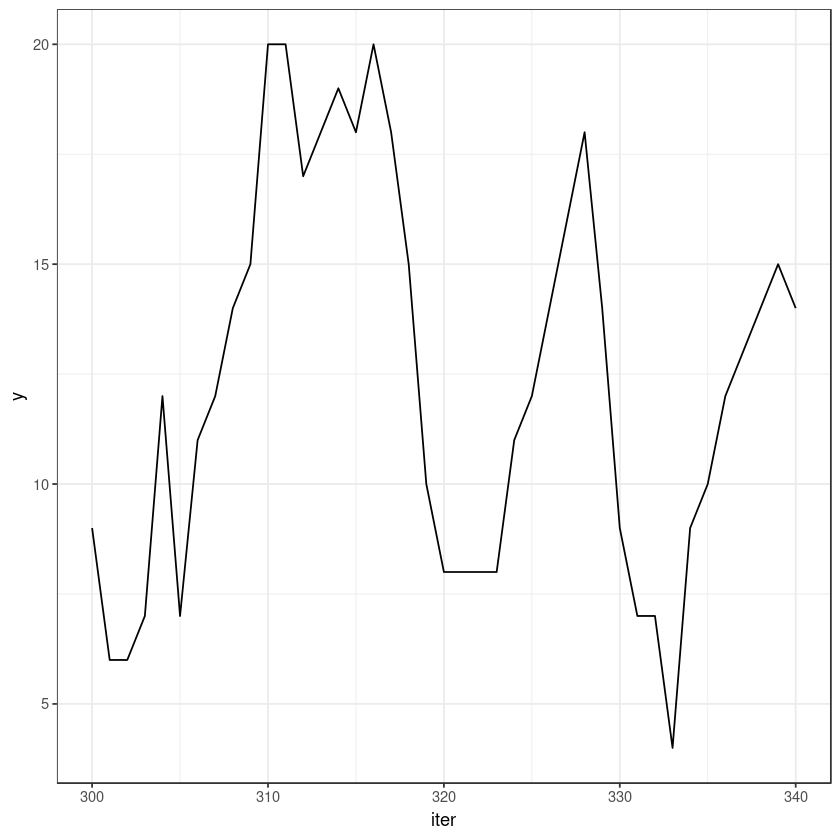Lets also take a look at convergence. Lets pick a rubbish starting value, and watch what happens early on: In : system.time(beta_binom_converge <- rand_BetaBinomial(1e5, 20, 3, 2, warmup = 1, theta_init = 0.0001))   user system elapsed 0.330 0.002 0.332  In : beta_binom_converge$iter <- 1:nrow(beta_binom_converge)
ggplot(beta_binom_converge[1:40,]) + geom_line(aes(iter, y))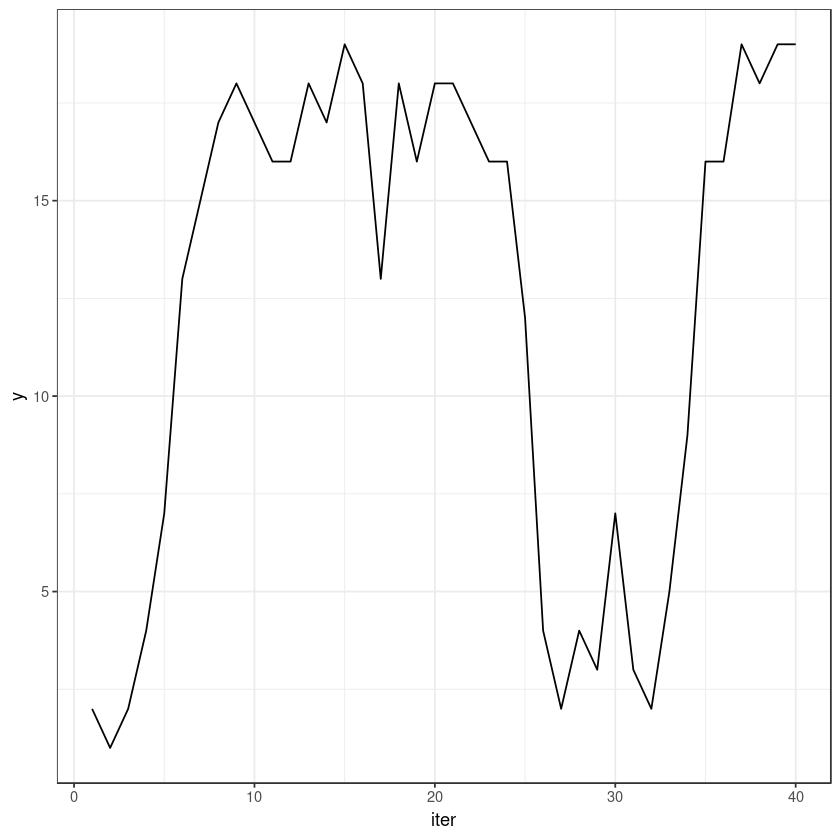In :
ggplot(beta_binom_converge[1:40,]) + geom_line(aes(iter, theta))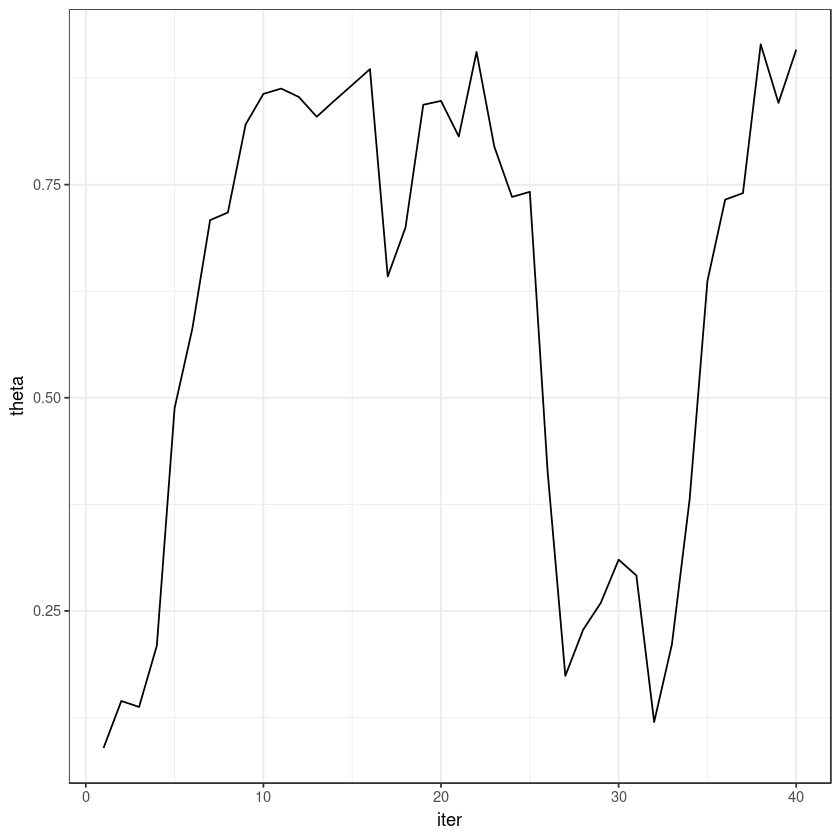Hopefully it is clear what is happening: the initial value of theta was close to 0, so that the first value of y was also small. A small sample of y leads to a small estimate for theta, to a small y…

But we can see it did not take long to get out of this rut. In fact, it converged very fast.
Within 10 iterations, $\theta$ and $y$ already exceeded their means of 0.6 and 12. The taint of our bad initial value is gone by 10 iterations: this model converges extremely quickly.
I made the function default to a warmup of 1000. In retrospect, that looks extremely excessive!

Finally, let’s run the model in JAGS for good measure.

In :
library(runjags)

beta_binomial_JAGS_model <- "model {
theta ~ dbeta(alpha, beta)
y ~ dbinom(theta, N)
}"

system.time(
beta_binom_JAGS <- run.jags(
beta_binomial_JAGS_model,
data = list(N = 20, alpha = 3, beta = 2),
monitor = c("theta", "y"),
n.chains = 1, burnin = 500, sample = 1e5
)
)
summary(beta_binom_JAGS)

Attaching package: ‘runjags’

The following object is masked from ‘package:tidyr’:

extract


Compiling rjags model...
Calling the simulation using the rjags method...
Note: the model did not require adaptation
Burning in the model for 500 iterations...
Running the model for 100000 iterations...
Simulation complete
Calculating summary statistics...

Warning message:
“Convergence cannot be assessed with only 1 chain”
Finished running the simulation

   user  system elapsed
0.553   0.142   0.722 
Lower95 Median Upper95 Mean SD Mode MCerr MC%ofSD SSeff AC.10 psrf
theta 0.2418793 0.611111 0.9614024 0.5979383 0.199277 NA 0.00199277 1 10000 0.001535863 NA
y 3.0000000 12.000000 19.0000000 11.9651000 4.462900 14 0.04399594 1 10290 0.001874215 NA

Lets update our old plot:

In :
binom_jags <- data.frame(beta_binom_JAGS$mcmc[]) jags_distribution <- binom_jags %>% select(y) %>% group_by(y) %>% tally() %>% mutate( probability = n / sum(n), estimate = rep("JAGS", length(n)) ) y_summary <- rbind(y_summary, jags_distribution) ggplot(y_summary) + geom_col(aes(y, probability, fill = estimate), position = position_dodge())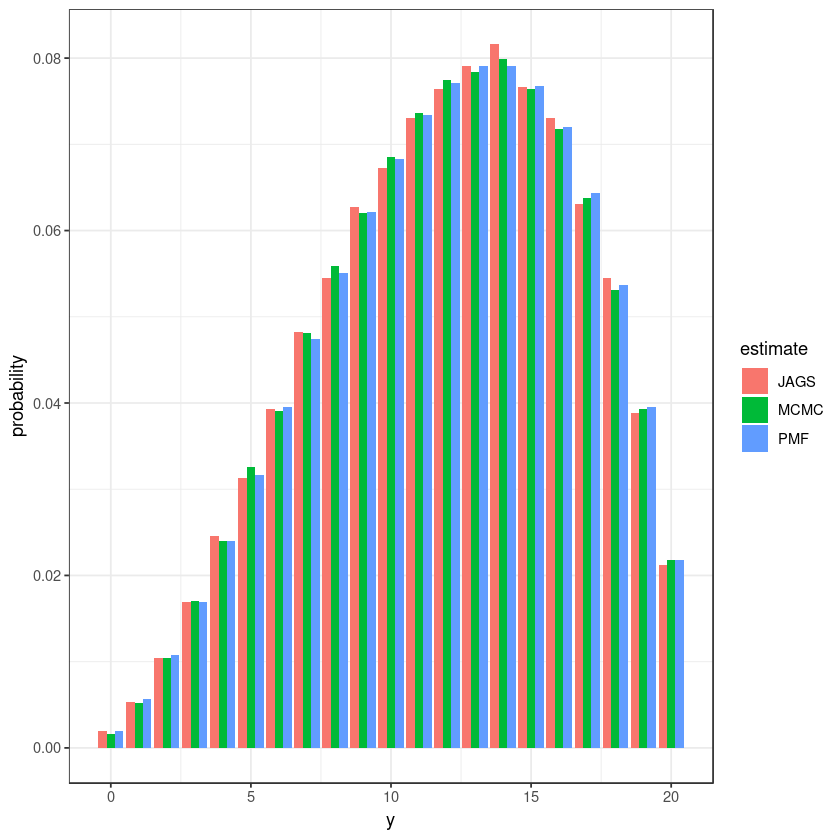Snazzy. Lets also see how JAG’s effective sample size estimate compares to our own: In : c( SS = nrow(binom_jags), ESS_y = effective_sample_size(binom_jags$y),
ESS_theta = effective_sample_size(binom_jags$theta) )  SS 1e+05 ESS_y 110837.406672068 ESS_theta 110506.375716026 Clever girl! Those effective sample sizes are as big as the actual sample sizes. Lets take a look at JAG’s autocorrelation: In : acf(binom_jags$theta)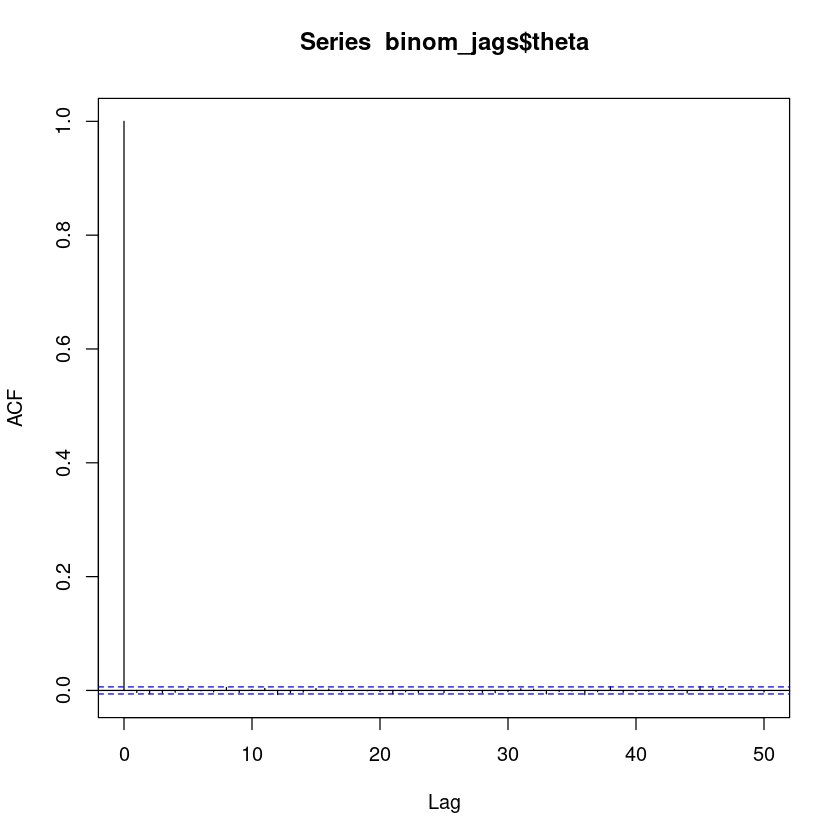Rather, the lack of it. Compare that to ours:

In :
acf(beta_binom$theta)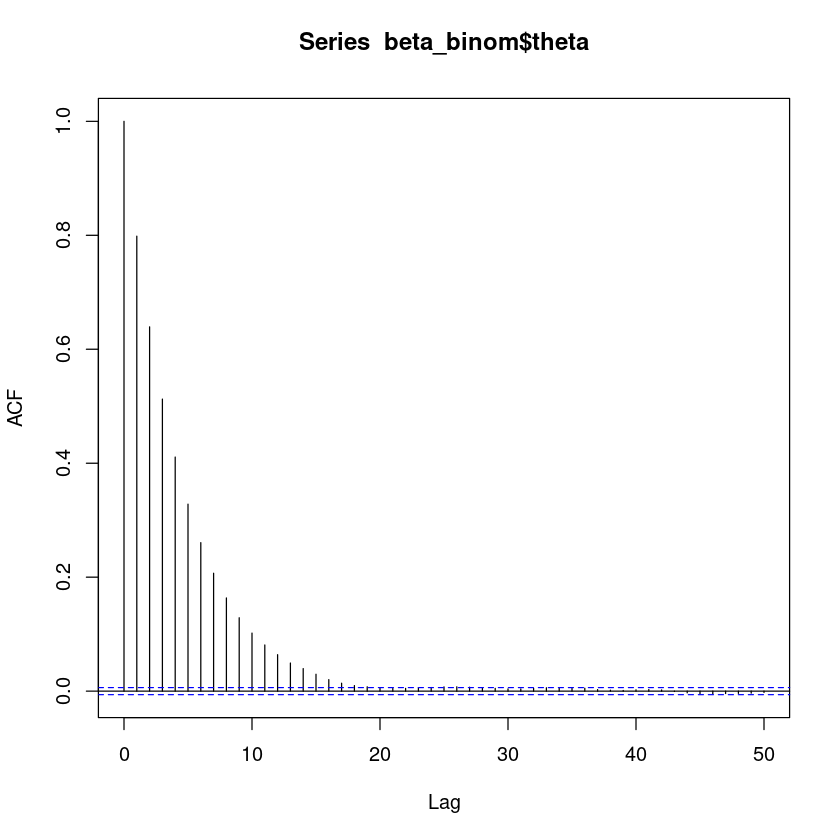It looks like JAGS wasn’t Gibbs sampling here at all. Instead, it identified the$\theta$-$y$pair as conjugate priors that it could sample from jointly, rather than iteratively sampling between them. That sort of trick can be a boon for performance (particularly in effective sample size), especially when a model contains a lot of conjugate pairs. But it only works when there are conjugate priors with a distribution JAGS recognizes. rmutil of course lets us sample from the BetaBinomial as well, but it only lets us sample the y. Doing that of course gives us zero autocorrelation as well: In : system.time(acf(rbetabinom(1e5, 20, 0.6, 5)))   user system elapsed 15.329 0.000 15.379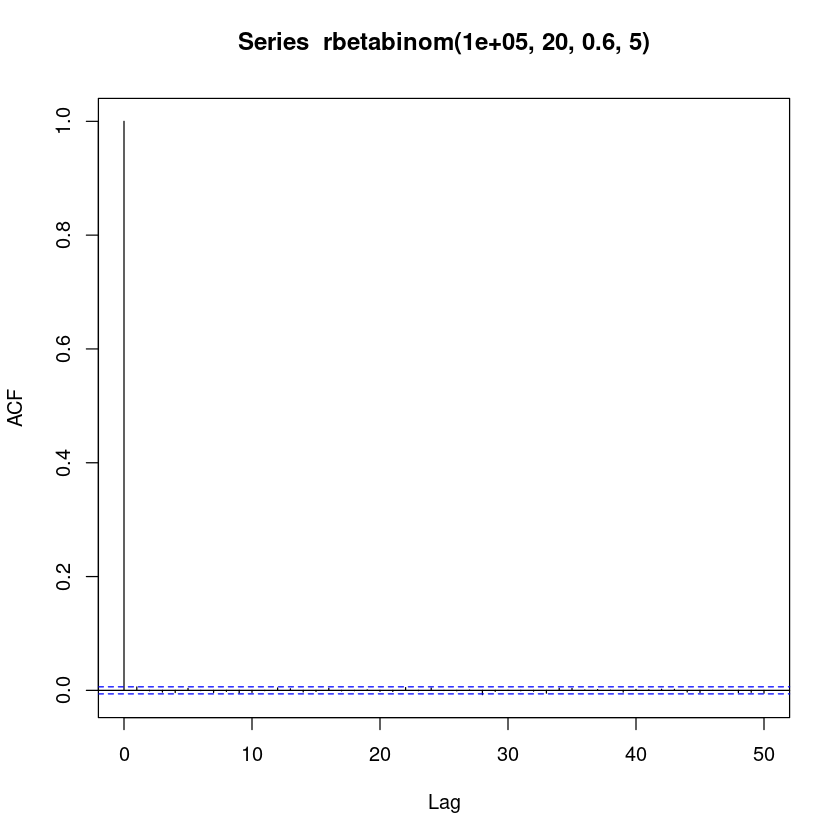Note how much faster JAGS was for the same number of samples, with the bonus of returning theta as well! And being far more flexible. JAGS is a solid piece of software. NIMBLE is probably similar, with the advantage of letting you define your own distributions and samplers for them, in case performance is important and you happen to know something NIMBLE does not$-\$ which could often be the case when you get creative.